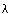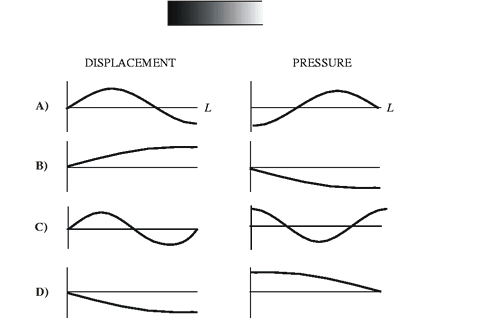TUTORIAL QUESTIONS 160-170

Questions:
160:  When standing waves are "flat."
165:  Fundamental frequency and wavelength.
170:  Displacement-pressure waves.

Question 160.  The time that elapses between any two successive instances where a standing sinusoidal wave on a string looks "flat" is

a) one period
b) 1/2 a period
c) 1/4 period
d) 1/4 or 3/4 period
e) always 2.589935 seconds, never more, never less.

Fundamentals of Sound reference: Sec. 2-A.

Question 165.  In a problem, if you have a way of computing a value for the fundamental frequency, f0, and the fundamental wavelength,0, for a sinusoidal standing wave on a string or an air column, then you can compute the value of any f (e.g., the ninth harmonic) or any(e.g., the wavelength of the twenty-third harmonic) for the higher harmonics. (Does the answer depend on boundary conditions?)

a) True
b) False

Fundamentals of Sound reference: Table III-1.

Question 170:  An air column with one end open and one end closed is vibrating in its first mode.  At a particular instant in time, the air molecules are distributed as in the picture below.  Which is the correct pair of displacement-pressure waves corresponding to this instant in time?A)   B)   C)   D)

Fundamentals of Sound reference: Sec. 3-D.

Back to Tutorial Listing